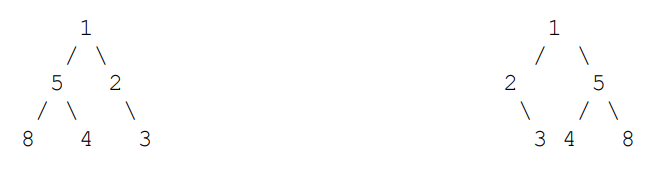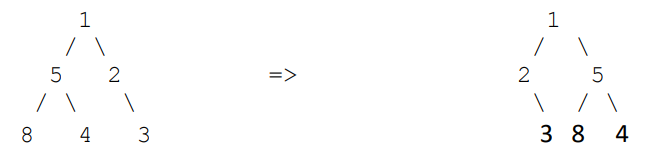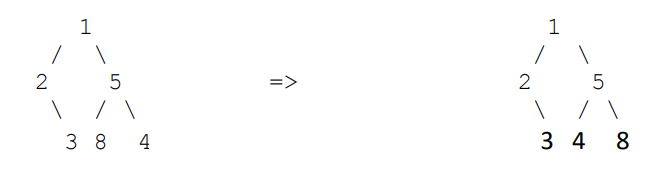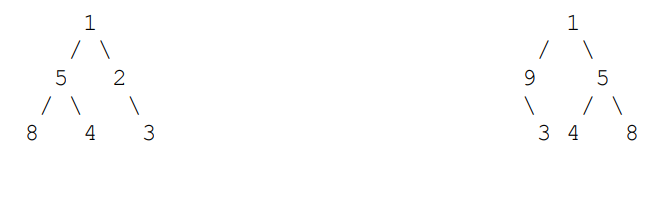# Check if Tree is Isomorphic

In this article, we are going to see how to check a tree whether isomorphic or not? This problem has been featured in interview coding round of Microsoft, Amazon.
Submitted by Radib Kar, on February 04, 2019

Problem statement:

Write a function to detect if two trees are isomorphic. Two trees are called isomorphic if one of them can be obtained from other by a series of flips, i.e. by swapping left and right children of a number of nodes. Any number of nodes at any level can have their children swapped.

Example1:These two trees are isomorphic

Swap left child & right child of 1Swap left & right child of 5Example 2:Solution:

The conditions which needed to be satisfied are:

1. Empty trees are isomorphic
2. Roots must be the same
3. Either left subtree & right subtree of one must be same with the same of other's, or left subtree of one must been same with right subtree of other's & right subtree of one must same with left subtree of other's.

Pre-requisite:

Two Input binary trees (their roots actually), i.e., root1, root2

```FUNCTION isIsomorphic(Node *root1,Node *root2)
1.  Both are empty then it's isomorphic. //condition-1
IF (!root1 && !root2)
return true;
If particularly exactly one of them is empty,
then they can't be isomorphic.//extension of condition-1
IF(!root1 || !root2)
return false;
2.  If root value are different, they can't be isomorphic//condition-2
IF(root1->data!=root2->data)
return false;
3.  Check condition-3
Recursively checking subtrees
return ( (  isIsomorphic(root1->left,root2->left) &&
isIsomorphic(root1->right,root2->right) ) ||
(isIsomorphic(root1->right,root2->left) &&
isIsomorphic(root1->left,root2->right)));
END FUNCTION
```

C++ implementation

```#include <bits/stdc++.h>
using namespace std;

// TreeNode node type
class TreeNode{
public:
int val;           //value
TreeNode *left;    //pointer to left child
TreeNode *right;   //pointer to right child
};

// creating new node
TreeNode* newnode(int data)
{
TreeNode* node = (TreeNode*)malloc(sizeof(TreeNode));
node->val = data;
node->left = NULL;
node->right = NULL;

return(node);
}

// function to check isomorphic trees
bool isIsomorphic(TreeNode *root1,TreeNode *root2)
{
if(!root1 && !root2)
return true;

if(!root1 || !root2)
return false;

if(root1->val!=root2->val)
return false;

return ( (isIsomorphic(root1->left,root2->left) &&
isIsomorphic(root1->right,root2->right) )||
(isIsomorphic(root1->right,root2->left) &&
isIsomorphic(root1->left,root2->right)));
}

int main(){
cout<<"tree is built as per example-1\n";

TreeNode *root1=newnode(1);
root1->left= newnode(5);
root1->right= newnode(2);
root1->right->right=newnode(3);
root1->left->left=newnode(8);
root1->left->right=newnode(4);

TreeNode *root2=newnode(1);
root2->left= newnode(2);
root2->right= newnode(5);
root2->right->right=newnode(8);
root2->right->left=newnode(4);
root2->left->right=newnode(3);

if(isIsomorphic(root1,root2))
cout<<"They are isomorphic tree\n";
else
cout<<"They are not isomorphic tree\n";

cout<<"tree is built as per example-2\n";

TreeNode *root3=newnode(1);
root3->left= newnode(9);
root3->right= newnode(2);
root3->right->right=newnode(3);
root3->left->left=newnode(8);
root3->left->right=newnode(4);

if(isIsomorphic(root1,root3))
cout<<"They are isomorphic tree\n";
else
cout<<"They are not isomorphic tree\n";

return 0;
}
```

Output

```tree is built as per example-1
They are isomorphic tree
tree is built as per example-2
They are not isomorphic tree
```

Explanation with example

Let's check the example-1

Nodes are represented by their respective values for better understanding

```In the main we call isIsomorphic(root1,root2) //isIsomorphic(1,1)
------------------------------------------------

isIsomorphic(1,1)
root1 is not NULL
root2 is not NULL
root1->data==root2->data
thus it returns
((isIsomorphic(root1->left,root2->left) && isIsomorphic(root1->right,root2->right)) ||
(isIsomorphic(root1->right,root2->left) && isIsomorphic(root1->left,root2->right)));
i.e.,
((isIsomorphic(5,2) && isIsomorphic(2,5)) ||
(isIsomorphic(2,2) && isIsomorphic(5,5)));

Thus call to isIsomorphic(5,2), isIsomorphic(2,5),
isIsomorphic(2,2), isIsomorphic(5,5)
------------------------------------------------

isIsomorphic(5,2)
root1 is not NULL
root2 is not NULL
root1->data!=root2->data
thus it returns FALSE
------------------------------------------------

isIsomorphic(2,5)
root1 is not NULL
root2 is not NULL
root1->data!=root2->data
thus it returns FALSE
------------------------------------------------

isIsomorphic(2,2)
root1 is not NULL
root2 is not NULL
root1->data==root2->data
thus it returns
((isIsomorphic(root1->left,root2->left) && isIsomorphic(root1->right,root2->right) ) ||
(isIsomorphic(root1->right,root2->left) && isIsomorphic(root1->left,root2->right)));
i.e.,
((isIsomorphic(NULL,NULL) && isIsomorphic(3,3)) ||
(isIsomorphic(3,NULL) && isIsomorphic(NULL,3)));

Thus call to isIsomorphic(NULL,NULL), isIsomorphic(3,3),
isIsomorphic(3,NULL), isIsomorphic(NULL,3)
------------------------------------------------

isIsomorphic(NULL,NULL)
root1 is NULL
root2 is NULL
thus it return TRUE
------------------------------------------------

isIsomorphic(3,3)
root1 is not NULL
root2 is not NULL
root1->data==root2->data
thus it returns
((isIsomorphic(root1->left,root2->left) && isIsomorphic(root1->right,root2->right) ) ||
(isIsomorphic(root1->right,root2->left) && isIsomorphic(root1->left,root2->right)));
i.e.,
((isIsomorphic(NULL,NULL) && (isIsomorphic(NULL,NULL) ||
(isIsomorphic(NULL,NULL) && (isIsomorphic(NULL,NULL)));

Thus call to isIsomorphic(NULL,NULL), (isIsomorphic(NULL,NULL),
(isIsomorphic(NULL,NULL), (isIsomorphic(NULL,NULL))

All (isIsomorphic(NULL,NULL) returns TRUE
Thus, isIsomorphic(3,3) returns TRUE
------------------------------------------------

isIsomorphic(3,NULL)
exactly one root is empty
Thus it returns FALSE
------------------------------------------------

isIsomorphic(NULL,3)
exactly one root is empty
Thus it returns FALSE

------------------------------------------------

Thus ,
isIsomorphic(2,2)
=((isIsomorphic(NULL,NULL) && isIsomorphic(3,3) ) ||
(isIsomorphic(3,NULL) && isIsomorphic(NULL,3)))
=(TRUE && TRUE)|| (FALSE && FALSE)
=TRUE && FLASE
=TRUE

Same way, we can check that isIsomorphic(5,5) returns TRUE
------------------------------------------------

At main:
isIsomorphic(1,1)
=((isIsomorphic(5,2) && isIsomorphic(2,5)) ||
(isIsomorphic(2,2) && isIsomorphic(5,5)))
=((FALSE && FALSE)||(TRUE && TRUE)
=(FALSE && TRUE)
TRUE

Thus these two trees are isomorphic.
```

You can check the second example same way & can find returning FALSE